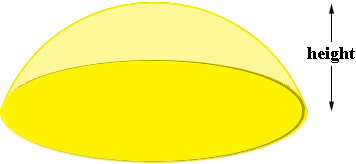SEARCH HOMEMath Central Quandaries & QueriesQuestion from Richard: I have to gold leaf a dome with a circumference of 29'. What would the exterior square footage be?Richard,

Is the dome is half a sphere? If so then, since the circumference of a circle is 2 π r where r is the radius, the radius of your sphere is 29/(2 × π) = 4.615 feet. The surface area of a sphere is 4 π r2 and hence in your situation that would be 4 π 4.6152 = 267.70 square feet. Thus half the sphere would have a surface area of 267.70/2 = 133.8 square feet.

If your dome is spherical but less then half a sphere tell us the height of the dome.PennyMath Central is supported by the University of Regina and The Pacific Institute for the Mathematical Sciences.